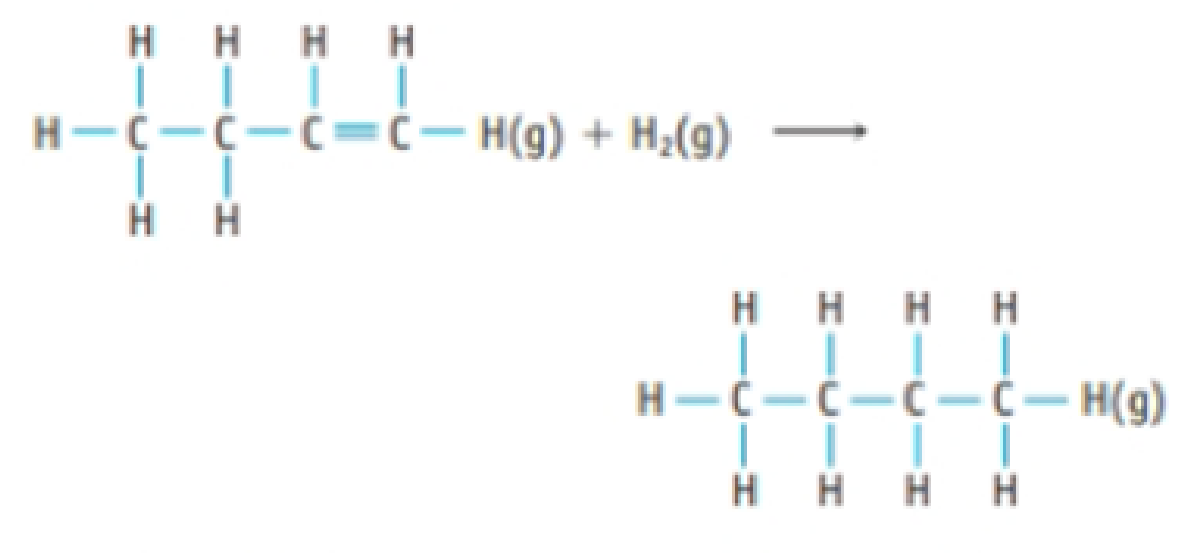Chapter 8, Problem 53PS

Chapter
Section
Textbook Problem

Hydrogenation reactions, which involve the addition of H2 to a molecule, are widely used in industry to transform one compound into another. For example, 1-butene (C4H8) is converted to butane (C4H10) by addition of H2.Use the bond dissociation enthalpies in Table 8.8 to estimate the enthalpy change for this hydrogenation reaction.

Interpretation Introduction

Interpretation: The enthalpy change for the given hydrogenation reaction should be determined.

Concept Introduction:

Bond dissociation enthalpy:

Bond energy or more correctly the bond dissociation enthalpy is the enthalpy change when breaking a bond in a molecule with the reactant and products in the gas phase.

Hydrogenation reaction:

Addition of hydrogen to a molecule is known as hydrogenation reaction.

Explanation

Given: 1-butene (C4H8) is converted to butane (C4H10) by adding H2 to it. The given reaction involves addition of hydrogen hence type of conversion is called Hydrogenation Reaction.

Using the bond dissociation enthalpy in table (8.8), we can estimate the enthalpy change for this hydrogenation reaction.

Consider the combustion of one mole of methane gas.

1-butene +H2butane

From table 8.8, Bond dissociation enthalpy for C-His413kJ/molC=Cis610kJ/mol and C-Cis346kJ/mol.

Examining the reaction shows that there are 1C=C and1H-H broken in reactant side and the bond formed in product sides are 1C-C and2

Still sussing out bartleby?

Check out a sample textbook solution.

See a sample solution

The Solution to Your Study Problems

Bartleby provides explanations to thousands of textbook problems written by our experts, many with advanced degrees!

Get Started